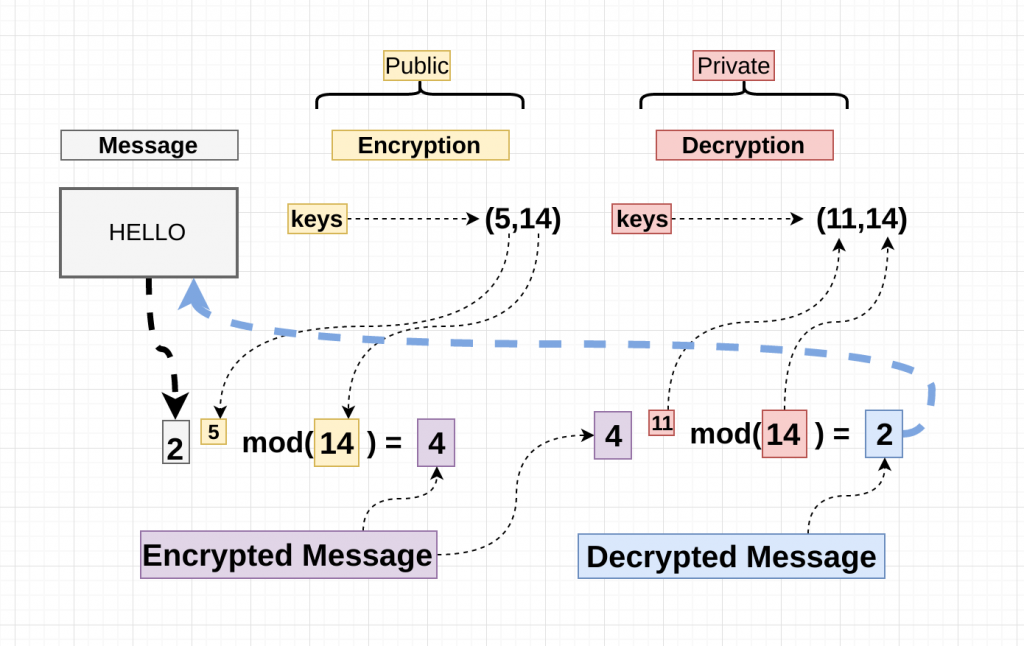#DAY 23
1

## 『 Day 23』密碼卷宗 現代篇 非對稱章 - RSA## RSA

• 安全基礎
• 質因數分解問題

### 基本數學

#### Euler φ function

• 又名尤拉函數歐拉函數

• φ(n)：比 n 小且與 n 互質的數

``````φ(4) = 2

``````
• 性質
• 如果 n 是質數：φ(n) = n - 1
• 如果 p 、 q 是質數：φ(pq) = φ(p) * φ(q) = (p-1)(q-1)
• 如果 p 是質數：φ(p^k) = p^k - p^(K-1)

#### mod ; 模運算

``````7 / 4 = 1 ... 3

7 mod 4 = 3

4 稱為「模數」
``````
• 性質

• (a mod p) ∗ (b mod p) = (a ∗ b) mod p
``````(5 mod 3) ∗ (7 mod 3) = (5 ∗ 7) mod 3 = 2
``````
• 除法

• 模運算中，看到除法要轉成乘法除數要改成在該模數下的模反元素
``````Example：

16 / 2 mod 3 = 2

16 ∗ invert(2,3) mod 3

16 * 2 mod 3 = 2

``````

#### mod inverse ; 模反元素

• 乘法反元素: 兩個數字相乘 = 1

``````Example：3 和 1/3 互為對方的乘法反元素
``````
• 模反元素: 兩個數字相乘後，除以模數的餘數 = 1

• 若 d 是 e 在模數是 \$φ\$(n) 下的模反元素
• e * d ≡ 1 (mod \$φ\$(n))
• e * d % \$φ\$(n) = 1
``````Example：e = 3 、 d = 5 、 \$φ\$(n) = 7
``````
• 求法

• 暴力窮舉
• 費馬小定理
• 擴展歐基里德演算法
• Python

``````from Crypto.Util.number import inverse
print(inverse(3, 7)) # 5
``````
``````import gmpy2 print(gmpy2.invert(3, 7)) # 5
``````

### 基本概念• 金鑰生成

• 選兩個質數 `p``q`

• `n = p * q`
• `φ(n) = (p-1) * (q-1)`
• 隨便找個數字 `e`

• `小於 φ(n)`
• `gcd(e, φ(n)) = 1`
• 透過 e 與 φ(n) 計算 d

• `e * d mod φ(n) = 1` (即 d 為 e 在 mod φ(n) 下之乘法反元素)
• `Public Key = (e, n)`

• `Private Key = d`

• 加密：C(密文) = P(明文)^e^ mod n

``````C = pow(M,e,N)
``````
• 解密：P(明文) = C(密文)^d^ mod n
``````M = pow(C,d,N)
``````

• 舉例
• P(明文)=2

• 已知 n = 33、e = 13 ，則

• p = 3 、 q = 11
• φ(n) = (3-1)x(11-1) = 20
• d = 17
• e * d mod φ(n) = 1
13 * d mod 20 = 1
當 (13 * n - 1) % 20 == 0
d = n
• 加密

• C = 2^13^ mod 33 = 8
• 解密

• P = 8^17^ mod 33 = 2